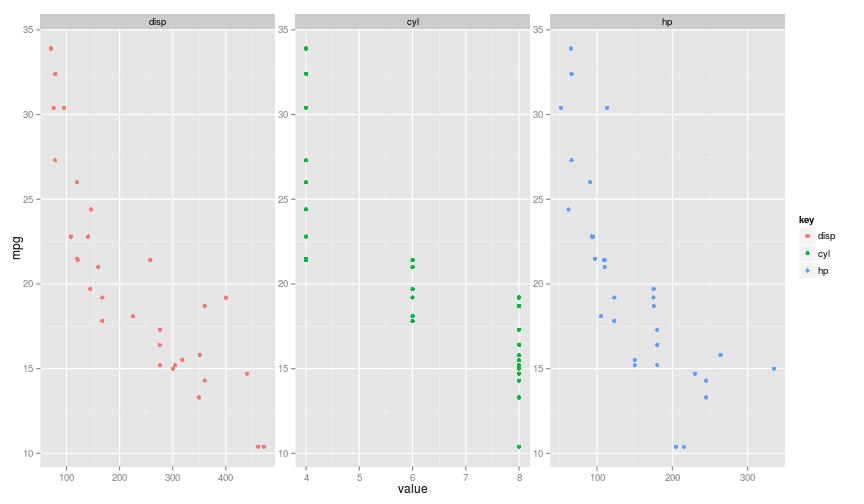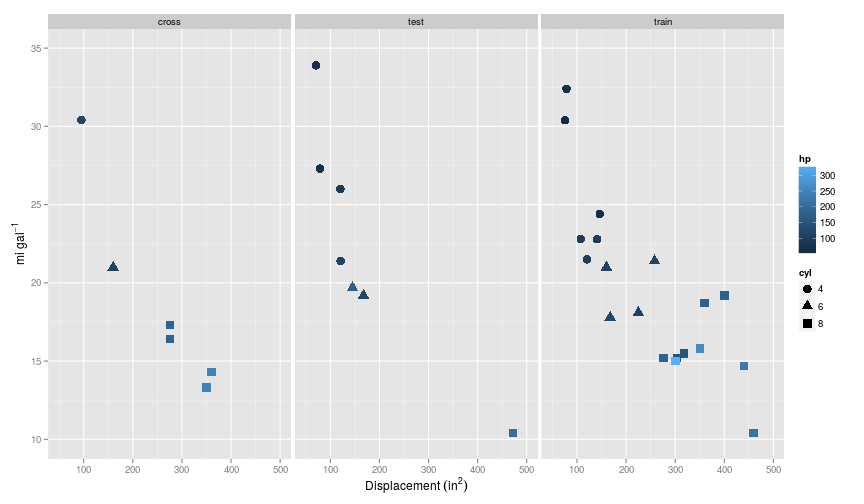Transponster

# Regularised linear regression

In this post I reproduce an example similar to an exercise I did for the coursera MOOC course in machine learning written by Andrew Ng. I’m compelting the course musing R, not the requisite matlab. In the next couple of posts I’m going to complete the equivalent of exercise 5.

The exercise was about creating a vectorised implementation of regularised linear regression, and using this to test some theory relating to the diagnosis of bias (underfitting) and variance (overfitting). I will use a different, but similar dataset to avoid publishing the solutions here.

### What is regularisation all about?

Regularisation helps us to deal with the problem of overfitting by reducing the weight given to a particular feature $x$. This allows us to retain more features while not giving undue weight to one in particular. Regularisation is mediated by a parameter $\lambda$, as can be seen in the cost function:

The first term is essentially the mean-squared-error term, whilst the additive term multiplies the sum of the square of the parameters ($\theta$) by $\lambda$ over $2m$, where $m$ is the number of training examples. Since the objective is to minimise $J(\theta)$ ($\underset{\theta}{\text{min}}J(\theta)$) using a large $\lambda$ will require small values of $\theta_j$ in order to acheive a minima.

### Getting some data

In this example I’m using data from the well worn mtcars dataset which is included in the datasets package, instead of the data given in the course. For the first example presented here, I limit myself to just the first five columns of this dataset, which are: mpg, cyl, disp, and hp, or: miles per gallon, number of cylinders, displacement ($\text{in}^2$), and gross horsepower (run ?mtcars for a data description). In this example, I want to predict mpg using cyl, disp, and hp as features.

Plotting each individually gives us a sense that they all have pretty correlations with mpg, but it’s also obvious that the features are correlated: e.g. disp and hp.We can also see that the range of values that the features take vary quite a lot. Whilst cyl $\leq{8}$, disp tends to measured in the hundreds of $\text{in}^2$.

There are 32 rows in the data set, and for this example I will make a 60/20/20 split on the dataset for train/cross-validate/test, giving 19 rows in the test set, and 6 and 7 in the cross-validation and test sets respectively.

At this point is has already become a little difficult to display all the features on a simple two dimensional plot, so I’ll use a combination of colour and shape.So each split of the data has retained some semblance of the curvature present in the training set.

### Regularised linear regression

To run the linear regression, I’ll build on the vectorised linear regression implementation I implemented here, but this time including a regularisation term.

A vectorised implementation of the cost function is given below. Note I’ve used tcrossprod(theta, X) as this function was about 1.5 times quicker than the equivalent X %*% theta in my tests, and both return the result of $\theta^TX$.

The cost function is not applied to $\theta_0$ as this relates to the intercept parameter.

The gradient function is given below, and is the same as that given in my previous post on regularised logistic regression Note that once again the regularisation term excludes $\theta_0$.

### Optimisation algorithm

As an optimisation algorithm, I’m use the optim function which ships with the stats package in vanilla R. Previously I used the ucminf function, and these two take the same arguments, so switching out the algorithms in the code is very simple. As default I have stuck to the BFGS method, and to $\lambda=0$, i.e. no regularisation.

I’ve also just included the parameters as they are, so any solutions are going to be strictly linear, and therefore many not be a very good fit.

So far so good, this seems to work: